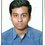If $a,b,c,d,e,f$ are positive integers and $\dfrac{a}{b}<\dfrac{c}{d}<\dfrac{e}{f}$.

Suppose $af-be=-1$ ,then prove that $d \geq b+f$.Note by Md Zuhair
4 years, 9 months ago

This discussion board is a place to discuss our Daily Challenges and the math and science related to those challenges. Explanations are more than just a solution — they should explain the steps and thinking strategies that you used to obtain the solution. Comments should further the discussion of math and science.

When posting on Brilliant:

• Use the emojis to react to an explanation, whether you're congratulating a job well done , or just really confused .
• Ask specific questions about the challenge or the steps in somebody's explanation. Well-posed questions can add a lot to the discussion, but posting "I don't understand!" doesn't help anyone.
• Try to contribute something new to the discussion, whether it is an extension, generalization or other idea related to the challenge.

MarkdownAppears as
*italics* or _italics_ italics
**bold** or __bold__ bold
- bulleted- list
• bulleted
• list
1. numbered2. list
1. numbered
2. list
Note: you must add a full line of space before and after lists for them to show up correctly
paragraph 1paragraph 2

paragraph 1

paragraph 2

[example link](https://brilliant.org)example link
> This is a quote
This is a quote
    # I indented these lines
# 4 spaces, and now they show
# up as a code block.

print "hello world"
# I indented these lines
# 4 spaces, and now they show
# up as a code block.

print "hello world"
MathAppears as
Remember to wrap math in $$ ... $$ or $ ... $ to ensure proper formatting.
2 \times 3 $2 \times 3$
2^{34} $2^{34}$
a_{i-1} $a_{i-1}$
\frac{2}{3} $\frac{2}{3}$
\sqrt{2} $\sqrt{2}$
\sum_{i=1}^3 $\sum_{i=1}^3$
\sin \theta $\sin \theta$
\boxed{123} $\boxed{123}$

Sort by:

$\dfrac{a}{b} < \dfrac{c}{d} < \dfrac{e}{f} ;~ af - be = -1 \\ \dfrac{a}{b} < \dfrac{c}{d} \\ ad < bc \\ \boxed{ad + x = bc} \\ \dfrac{c}{d} < \dfrac{e}{f} \\ cf < de \\ \boxed{cf + y = de\\ cf = de - y} \\ \boxed{af - be = -1 \\ af = be-1}\\ ad + x = bc \\ adf + xf = bcf \\ d(af) + xf = b(cf) \\ d(be-1) + xf = b(de-y) \\ dbe - d + xf = dbe - by \\ xf - d = -by \\ d = xf + by \\ \boxed{d \ge b + f}$

- 4 years, 9 months ago

You are going to top TN Region RMO This time @Vicky Vignesh

- 4 years, 9 months ago

Great! The interesting fact is that $d = xf + by$, which is extremely restrictive.

Staff - 4 years, 9 months ago

Yeah, restricts $x, y \in R$

- 4 years, 9 months ago

What have you tried? What do you know?

Staff - 4 years, 9 months ago

- 4 years, 9 months ago

How have you tried to solve this problem?

For example, do you know if $d > b$ or $d > f$?

Staff - 4 years, 9 months ago

I could'nt do it.

- 4 years, 9 months ago

@Vicky Vignesh .. Could you?

- 4 years, 9 months ago

What have you tried?

If you've tried nothing, then go try something. What approaches can you think of? What comparisons can we make?

Staff - 4 years, 9 months ago

I started by assuming d<b+f. Then I did lot of manipulations and after a while a wrong result came up which means this is a contradiction. So d must be >=b+f. Is this correct?

- 4 years, 9 months ago

Assuming that the steps are correct, then that sounds like a possible proof approach. You should write up the solution and others can comment on it.

Staff - 4 years, 9 months ago

You must use the given equality with the inequality. Try adding any variable to rest of inequality with a'equal to' symbol

- 4 years, 9 months ago

- 4 years, 4 months ago

Very nicely done. What is the motivation / intuition behind how you arrived at this solution? What led you to think about it?

Staff - 4 years, 4 months ago

It is easy to get bc-ad>0.b,c,a,d,e,f are integers.So I was wondering how can I use it in the problem?Then I thought that bc-ad>=1 might help as after all I have to show something >= something.So using bc-ad>=1 is better than using bc-ad>0.Hence I used it.Now I broke (dbe - bfc) in the following way so as to use the fact bc-ad>=1 & de-fc>=1 & finally arrived the solution.

- 4 years, 4 months ago

Wonderful! Thanks for explaining. This helps to demystify what you did. Previously when stated as 1 sentence, it seems like "I magically created this equation and got the answer".

You've now added "Here is my reasoning for why I considered these steps, and how I was wanting to use the conditions of the problem". This will help @Md Zuhair (and everyone else) learn from this and apply it to other scenarios.

Staff - 4 years, 4 months ago

Well, I couldnt do this :P

- 4 years, 4 months ago